# A cheatsheet of mathematical formulas for fundations of Continuous OptimizationGradient descent being like… — Photo by Alex Lange — Unsplash

A big part of the above formulas are from my notes at the DSTI. I also added curves I found or created, as well as more gradient descent algorithms. Please note that it is a formulas cheat sheet, not a course. It is good to check or refresh your knowledge.

If you are specifically interested in gradient descent algorithms and not so much into refreshing mathematical foundations such as Euler equality or the Lagrangian, you may like the following article, that dive more into the details of each optimization algorithms: “A glimpse of the maths behind Optimization Algorithms”.

• Indexes : 1, 2, … , i, j, k, m, n ∈ [1,n] ⊂ ℕ
• In regular: a, b, c, …, t, u, v, x, y, z ∈ ℝ
• In bold: x is a vector of coordinates xᵢ ∈ ℝ with i ∈ [1,n]
• Usually, x is the variable, y and z directions of space. While a and b are fixed vectors.
• êᵢ are the unit vectors of the orthonormal basis
• In uppercase: A is a matrix of size n×m and elements aᵢⱼ
• J : V ⊂ ℝⁿ ↦ ℝ is the function to optimize
• ε represent a small quantity in ℝ
• θ ∈ [0,1] ⊂ ℝ
• g is a vector of the gᵢ constraints such that g(x)=0 : ∀i ∈ [1, …,m₁]
• 𝛌 is the associated vectors of Lagrange multipliers λᵢ
• h is a vector of the hⱼ constraints such that hⱼ(x)≤0 : ∀j ∈ [1, …,m₂]
• 𝛍 is the associated vectors of Lagrange multipliers µⱼ

# What is a minimum?

x* is a global minimum of J if J(x*) ≤ J(x) ∀x :

x* is a local minimum of J if there is a neighbourhood N of x* where it is the minimum:

# Gateaux differentiability

J is Gateaux differentiable at x if:

• J’(x;y) exists ∀y
• y ↦ J’(x;y) is linear

If y is a vector êᵢ of the orthonormal basis:

We can write it with the gradient:

J is twice Gateaux differentiable at x if:

• J’’(x;y,z) exists ∀y,z
• (y,z) ↦ J’’(x;y,z) is bilinear

Using vectors of the orthonormal basis:

We can write it with the Hessian matrix:

Similarly to what we know for the second order partial derivatives:

At some occasions, we will use the first terms of the polynomial approximation of J in dimension n, in forms such as:

Also:

We can also use:

# Existence of a minimum

J : K ⊂ ℝⁿ → ℝ has a minimum if K is closed and either:

• K is bounded (what mean that K has a boundary and the boundary is included in K. We can also note that bounded + closed = compact)
• Or lim J(x) [when ∥x∥ → ] =

These examples, show why a closed set is part of the sufficient conditions, yet is not necessary: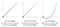Own work released under CC BY 3.0 — Download source

These examples, show why a bounded set or an infinite at infinite function is part of the sufficient conditions, yet is not necessary:Own work released under CC BY 3.0 — Download source

# Convexity

Since we usually want to minimize functions, convexity is a nice thing to check.

• If J is convex, any local minimum is the global minimum.
• If J is strictly convex, there exist only one minimum.

For all the above cases: ∀x,y ∈ K ; ∀θ ∈ [0,1]; α≥0

The set K ⊂ ℝⁿ is convex if the segment:

We need to have K convex, to have J: K → ℝ convex.

On ℝ², drawing potatoes K: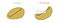Own work released under CC BY 3.0 — Download source

If K = ℝ, a counter-example could be {[0,1],[3,4]}, that is not convex, as any disjoint set.

alpha convex ⇒ strictly convex ⇒ convex

Graphically, this can be interpreted as: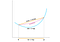Own work released under CC BY 3.0 — Download source

Or, “saying” that the direction of the tangent is increasing:

ヽ(⌐■_■)ノ♪♬

Actually, due to an issue at limits, J(x;y,y) >0 only ⇒ that J is strictly convex, while the others are ⇔ (but we will rarely run into it…).

We can rewrite this with the Eigenvalues λᵢ of the Hessian matrix: ∇²J(x):

# Optimality conditions

“How to find the min”

• K ⊂ ℝⁿ is open
• J is Gateaux differentiable

If K and J are convex, we even have an ⇔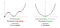Own work released under CC BY 3.0 — Download source

Second order, adding J twice G-derivable:

Sufficient condition, adding on a ball B(x*,ε):

The ball somehow define a “locally convex” function, around the minimum.

• K ⊂ ℝⁿ is convex
• J is Gateaux differentiable

If J is convex, we even have an ⇔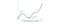Own work released under CC BY 3.0 — Download source

# Equality constraints

We search for inf J(x) ∀x ∈ K where K = { x ∈ X s.t. G(x) = 0 }, what mean for function g’s coordinates: gᵢ(x) = 0 ∀i ∈ {1,…,m}.

We say that the constraints g(x)=0 are regular at point x₀ if their derivatives are linearly independants.

If x* is a local minimum of J on K and if the constraints are regular at x*, then, there exists m real values λᵢ such that:

These values λᵢ are called Lagrange multipliers. There is a unique set of vectors that satisfy this relation.

Actually, we are saying that the derivative of a Lagrangian according to x is equal to zero at x*. So, with λ =(λ₁, …, λₘ), we associate the following Lagrangian to the minimization problem:

If we want to minimize both on J and on the regular constraints, writing the previous minimization problem with the Lagrangian gives:

The couple (x*,λ*) is a critical point of the lagrangian.

# Notion of saddle point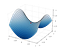By Nicoguaro — Wikipedia — CC BY 3.0

Let’s consider a fonction ℒ : ℝⁿ×ℝᵐ → ℝ

If (x*,y*) ∈ ℝⁿ×ℝᵐ is a saddle point of ℒ then:

# Inequality constraints

Similarly to equality constraints:

The inequality constraint implies that all the coordinates of the lagrange multiplier must be superior to zero. We will work with 𝛍 on the positive cone ℝᵐ₊:

If (x*,𝛍*) ∈ ℝⁿ×ℝᵐ is a saddle point of ℒ, then it belong to K and is a solution to the minimization problem with inequality constraints. We can write it as:

We can eliminate the J(x*) terms on both side:

There is 2 possible cases for each i:

• Either the constraint is inactive, what mean that x* is not on its boundary. Then we have μᵢ = 0 and hᵢ(x*) < 0.
• Either the constraint is active, what mean that x* is on the boundary. Then we have μᵢ ≥ 0 and hᵢ(x*) = 0.

In practice, this is how we will proceed. For each i, we will solve for both cases: μᵢ = 0 and hᵢ(x*) = 0.

This can be a lot of cases. If there is there 3 constraints (m=3), there is 2³ = 8 cases to solve.

We can rely on the Euler equality to solve it:

# Karush-Kuhn-Tucker

In the convex case, we can rely on the Karush-Kuhn-Tucker conditions, what makes things much easier. This necessary and sufficient conditions of optimality, given J convex, h and J derivables.

In the non-convex case, theses are a not sufficient yet necessary conditions for x* being a solution of the optimization with inequality problem.

If ∀i ∈ {1,…,m} ; ∃x* ∈ ℝ such that:

• either hᵢ(x*) < 0
• or: hᵢ(x*) = 0 with F affine.

Then x* is a solution of the minium with inequality constraints problem is equivalent to that, there exists 𝛍* such that (x*,𝛍*) that is a saddle point of ℒ on ℝⁿ×ℝᵐ₊.

We can write the Kuhn-Tucker relations:

• The 3 first lines are given by the left inequality of the saddle point
• The last line is given by the right inequality of the saddle point

We can also note that affine constraints are always qualified if there is only affine constraints and if the resulting subset in not empty.

We can write this case as:

We need 2 conditions, that may be pretty hard to check, except if all the constraints are affine.

Qualification hypothesis 1:

We need the existence of a vector z that permits the re-entrance in the domain of the constraints if we are at the boundary.

The second line may be an issue and shall be taken care of if some constraints are affine and some other are not affine.

Qualification hypothesis 2:

The gradients ∇gᵢ(x*) must be linearly independent ∀i ∈ {1,…,m₁}.

If x* is a local minimum of J on K and if the above qualification hypothesis are satisfied, then:

• ∃𝛌 = (λ₁, …, λₙ) ∈ ℝᵐ
• ∃𝛍 = (μ₁, …, μₘ) ∈ ℝᵐ¹₊

such that, ∀i ∈ {1,…,m₁} ; ∀j ∈ {1,…,m₂}:

This can also be written with matrices:

Practically, in an algorithm, when the hypothesis are hard to verify, we can check the value on the specific points where calculus issues arise.

# Base formulas of optimization algorithms

This part is only a list of formulas for reminder. If you want to get through the details and explanations you may read the article: “A glimpse of the maths behind Optimization Algorithms”.

The general formula for gradient descent is:

We are basically saying that we will move towards the opposite direction of the gradient by a factor ρₖ of the gradient norm.

In practice, terms are added to help it converve better and faster.

This is the most “mathematically simple” algorithm, while not a very performant one. Here, we simply choose a fixed value of ρₖ, that we will therefore call ρ.

We are working under the previous hypothesis. J is alpha-convex and Gateaux derivable.

J(xₖ) is Lipschitz continuous:

The condition for convergence is:

In this algorithm, we will also maximize the progress value of the step: J(xₖ₊₁)-J(xₖ), to find out the optimal value of rho.

The optimal step gradient algorithm converges for any J that is: Gateaux-derivable, Alpha-convex, Lipschitz continuous, on every bounded subset.

The algorithm converges in n step, where n is the dimension of the space. In practice rounding errors prevents the exact convergence in simulations, yet it remain faster than previous methods.

On quadratic functions, if we take dᵢ the direction of the gradient at the iᵗʰ iteration, the algorithm is the following:

One of the common uses of this algorithm is to solve linear systems in the form Ax = b, by transforming it into a quadratic function.

We need to solve:

In practice, since computing the Hessian is costly, we do it only at some steps.

With restrict the set on which we are looking for the minimum and call it K.

When xₖ is not on K, we will make a projection of the result to K:

J: ℝⁿ → ℝ is the function to minimize and F: ℝⁿ → ℝᵐ is the vector function of the constraints such that F(x)=0. We introduce a cost function:

Then we can solve on ℝⁿ, since any point out of {x s.t. F(x)=0} will be strongly penalized.

In practice, if epsilon is too small the problem become ill conditioned.

this is once again the fixed step gradient algorithm, but we inject a Lagrangian in the method. J is the function to minimize. F(x) is the vector of the conditions. J and F are considered as convex.

Uzawa algorithm can be quite slow. We can also add a penalty term called augmented Lagrangian that is square, alpha-convex and converge better than linear terms.

With stochastic gradient descent, at each step, you take a random subset of the data to compute the gradient. This makes gradient descent very noisy yet scalable on big data sets.

Similarly, we cut the data into a finite number of batches and rotate the computation of the gradient on each batch.

Momentum adds a fraction of the previous update vector to the current one. This can be written:

γ can be quite high, as 0.9 is a common value.

Here, we compute the gradient on actual position of point after adding the fraction of the previous update vector, rather than on the original starting point:

The idea of Adagrad is to:

• associate a lower learning rate to frequent features,
• associate a higher learning rate to more rare features.

Adagrad it therefore especially relevant with sparse datasets where some features occur rarely and carry lots of sense.

Adagrad defines a diagonal matrix Gₖ, that takes the sum of the subsequent gradients in its diagonal elements. It is used to apply a decay on the learning rate over time:

The weakness of Adagrad is that the accumulation term Gₖ continue to grow over time, eventually resulting in a very small learning rate. These methods intent to fix that by taking an exponentially decaying average of the squared gradients.

RMS Prop equations look like this:

Once again, beta can be quite high as a classical value is 0.9.

Adadelta even add an exponentially moving average of the squared update vectors:

Adam is inspired from AdaGrad and RMSProp. It is an often used general purpose optimizer.

Adams uses the first moment (expected value) mₖ and the second moment (variance) vₖ estimates of the gradient.

However, since m and v and initiated at 0 and since the β₁ and β₂ constants are near to 1, these terms are biased towards zero. Then, Adam compute a bias-corrected moment estimate.

Then, we can write Adam’s update function as:

There is many Adam-like algorithms that intent to improve its performance. There is also a trend towards parameters auto tuning over time, that seems promiseful.

Publications in French & English about design, innovation and programming.

## More from Thibaut

Publications in French & English about design, innovation and programming.